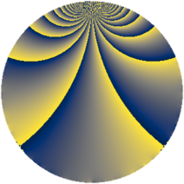Properties

 Label 1911.1.wLevel $1911$ Weight $1$ Character orbit 1911.w Rep. character $\chi_{1911}(116,\cdot)$ Character field $\Q(\zeta_{6})$ Dimension $28$ Newform subspaces $6$ Sturm bound $261$ Trace bound $3$

Related objects

Defining parameters

 Level: $$N$$ $$=$$ $$1911 = 3 \cdot 7^{2} \cdot 13$$ Weight: $$k$$ $$=$$ $$1$$ Character orbit: $$[\chi]$$ $$=$$ 1911.w (of order $$6$$ and degree $$2$$) Character conductor: $$\operatorname{cond}(\chi)$$ $$=$$ $$273$$ Character field: $$\Q(\zeta_{6})$$ Newform subspaces: $$6$$ Sturm bound: $$261$$ Trace bound: $$3$$

Dimensions

The following table gives the dimensions of various subspaces of $$M_{1}(1911, [\chi])$$.

Total New Old
Modular forms 60 44 16
Cusp forms 28 28 0
Eisenstein series 32 16 16

The following table gives the dimensions of subspaces with specified projective image type.

$$D_n$$ $$A_4$$ $$S_4$$ $$A_5$$
Dimension 28 0 0 0

Trace form

 $$28 q - 10 q^{4} - 14 q^{9} + O(q^{10})$$ $$28 q - 10 q^{4} - 14 q^{9} - 6 q^{16} - 16 q^{22} - 10 q^{25} + 8 q^{30} + 20 q^{36} + 2 q^{39} - 8 q^{43} + 4 q^{64} + 4 q^{79} - 14 q^{81} + 16 q^{88} + O(q^{100})$$

Decomposition of $$S_{1}^{\mathrm{new}}(1911, [\chi])$$ into newform subspaces

Label Dim. $$A$$ Field Image CM RM Traces $q$-expansion
$a_{2}$ $a_{3}$ $a_{5}$ $a_{7}$
1911.1.w.a $2$ $0.954$ $$\Q(\sqrt{-3})$$ $D_{2}$ $$\Q(\sqrt{-3})$$, $$\Q(\sqrt{-39})$$ $$\Q(\sqrt{13})$$ $$0$$ $$-1$$ $$0$$ $$0$$ $$q-\zeta_{6}q^{3}+\zeta_{6}q^{4}+\zeta_{6}^{2}q^{9}-\zeta_{6}^{2}q^{12}+\cdots$$
1911.1.w.b $2$ $0.954$ $$\Q(\sqrt{-3})$$ $D_{2}$ $$\Q(\sqrt{-3})$$, $$\Q(\sqrt{-39})$$ $$\Q(\sqrt{13})$$ $$0$$ $$1$$ $$0$$ $$0$$ $$q+\zeta_{6}q^{3}+\zeta_{6}q^{4}+\zeta_{6}^{2}q^{9}+\zeta_{6}^{2}q^{12}+\cdots$$
1911.1.w.c $4$ $0.954$ $$\Q(\sqrt{2}, \sqrt{-3})$$ $D_{4}$ $$\Q(\sqrt{-39})$$ None $$0$$ $$-2$$ $$0$$ $$0$$ $$q-\beta _{1}q^{2}+\beta _{2}q^{3}+\beta _{2}q^{4}+\beta _{1}q^{5}+\cdots$$
1911.1.w.d $4$ $0.954$ $$\Q(\sqrt{2}, \sqrt{-3})$$ $D_{4}$ $$\Q(\sqrt{-39})$$ None $$0$$ $$2$$ $$0$$ $$0$$ $$q-\beta _{1}q^{2}-\beta _{2}q^{3}+\beta _{2}q^{4}-\beta _{1}q^{5}+\cdots$$
1911.1.w.e $8$ $0.954$ 8.0.339738624.2 $D_{8}$ $$\Q(\sqrt{-39})$$ None $$0$$ $$-4$$ $$0$$ $$0$$ $$q+\beta _{3}q^{2}+\beta _{5}q^{3}+(\beta _{5}-\beta _{6})q^{4}+\beta _{1}q^{5}+\cdots$$
1911.1.w.f $8$ $0.954$ 8.0.339738624.2 $D_{8}$ $$\Q(\sqrt{-39})$$ None $$0$$ $$4$$ $$0$$ $$0$$ $$q+\beta _{3}q^{2}-\beta _{5}q^{3}+(\beta _{5}-\beta _{6})q^{4}-\beta _{1}q^{5}+\cdots$$